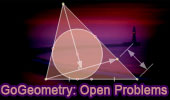# Plane Geometry: Open Problems, High School, CollegeOpen Geometry Problems - Index 2 Geometry Problem 1361. Post a solution Triangle, Two Nine-Point Circles, Feuerbach's Circle, Euler's Circle, Congruent Angles, Sketch, iPad Apps. Geometry Problem 1351. Post a solutionTwo Tangential or Circumscribed Quadrilaterals, Midpoints, Perpendiculars. Geometry Problem 1345 Post a solutionThree Equilateral Triangles, Center, Area, Common Vertex. Geometry Problem 1325. Post a solutionOctagons, Midpoints, Squares, Mobile Apps, iPad. Geometry Problem 1316. Post a solutionTriangle, Incircle, Tangent, Chord, Midpoint, Sum of two Segments, Congruence. Geometry Problem 1282. Post a solution Three Squares, Common Vertex, Midpoint, Perpendicular, 90 Degrees. Geometry Problem 1253. Post a solutionTriangle, Orthocenter, Circle, Circumcircle, Altitude, Perpendicular, 90 Degree, Concurrency. Geometry Problem 1238 Post a solutionSquare, Equilateral Triangle, Metric Relations. Mobile Apps. Geometry Problem 1233 Post a solutionTriangle, Euler Line, Orthic Axis, Perpendicular, 90 Degrees, Orthic Triangle. Geometry Problem 1212 Post a solution Equilateral Triangle, Equilateral Hexagon, Concurrent Lines. Geometry Problem 1210 Post a solution Circle, Tangent Line, Secant, Chord, Collinear Points. Geometry Problem 1176 Post a solutionCyclic Quadrilateral, Diagonals, Six Diameters, Circles, Collinear Points, Concurrent Lines. Geometry Problem 1168 Post a solutionConstruction of the Inscribed Circle of the Arbelos, Semicircles, Diameter, Circle, Triangle, Circumcircle, Tangent. Geometry Problem 1143. Post a solutionTriangle, Circumcircle, Tangent Line, Reflection of a Point over a Line, Tangent Circles, Collinear Points. Go to Page: Previous | 1 | 5 | Next

Home | Sitemap | Search | Geometry | Problems | All Problems | Ten Geometry Problems | Visual IndexEmail | By Antonio Gutierrez
Last updated: Mar 9, 2022